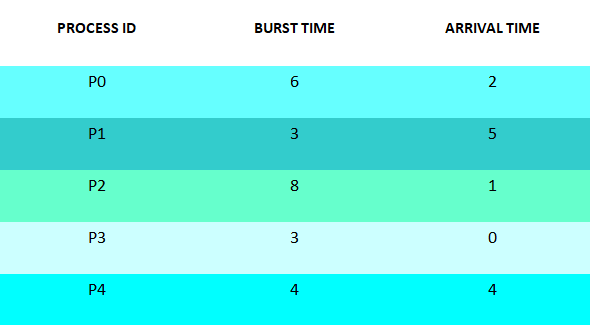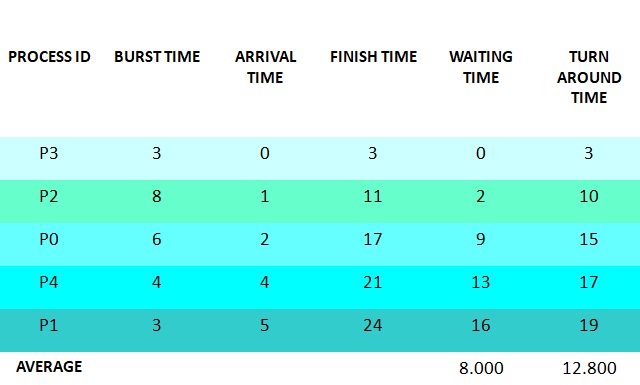×

Search anything:

# First Come First Serve CPU Scheduling Algorithm

#### Software Engineering AlgorithmsReading time: 30 minutes | Coding time: 15 minutes

CPU Scheduling algorithms are used for scheduling different processes present in the ready queue with available resources (CPU cores) in an optimal way so that each and every process get executed by CPU. Scheduling algorithms are broadly classified into two main types namely Preemptive and Non-preemptive.First Come First Serve is an Non-preemptive Scheduling algorithm where each process is executed according to its arrival time.

First Come First Serve (FCFS) is also known as First In First Out (FIFO) scheduling algorithm is the easiest and simplest CPU scheduling algorithm where the process which arrives first in the ready queue is executed first by the CPU. New process is executed only when the current process is executed fully by the CPU.

# Algorithm

• Step 1 : Input the number of processes required to be scheduled using FCFS, burst time for each process and its arrival time.
• Step 2 : Using enhanced bubble sort technique, sort the all given processes in ascending order according to arrival time in a ready queue.
• Step 3 : Calculate the Finish Time, Turn Around Time and Waiting Time for each process which in turn help to calculate Average Waiting Time and Average Turn Around Time required by CPU to schedule given set of process using FCFS.
• Step 3.1 : for i = 0, Finish Time T 0 = Arrival Time T 0 + Burst Time T 0
• Step 3.2 : for i >= 1, Finish Time T i = Burst Time T i + Finish Time T i - 1
• Step 3.3 : for i = 0, Turn Around Time T 0 = Finish Time T 0 - Arrival Time T 0
• Step 3.4 : for i >= 1, Turn Around Time T i = Finish Time T i - Arrival Time T i
• Step 3.5 : for i = 0, Waiting Time T 0 = Turn Around Time T 0 - Burst Time T 0
• Step 3.6 : for i >= 1, Waiting Time T i = Turn Around Time T i - Burst Time T i - 1
• Step 4 : Process with less arrival time comes first and gets scheduled first by the CPU.
• Step 5 : Calculate the Average Waiting Time and Average Turn Around Time.
• Step 6 : Stop.

### Example of First Come First Serve Algorithm

Consider the following example containing five process with varied arrival time.• Step 1 : Processes get executed according to their arrival time.
• Step 2 : Following shows the scheduling and execution of processes.
• Step 2.1 : At start P3 arrives and get executed because its arrival time is 0. Its duration of execution is 0-3 seconds.
``````System Time : 0
Process Scheduled  : P3
Waiting Time       : 3 – 3 = 0
Turn Around Time   : 3 - 0 = 3
``````
• Step 2.2 : P2 arrives at time 1 sec during which CPU was busy with Process P3.After completion of P3 , P2 is executed for duration 3-11 seconds.
``````System Time        : 3
Process Scheduled  : P3 P2
Waiting Time       : 10 – 8 = 2
Turn Around Time   : 11 – 1 = 10
``````
• Step 2.3 : P0 arrives at time 2 sec but its execution is started at 11 sec after complete execution of process P2 , for a duration 11-17 seconds.
``````System Time        : 11
Process Scheduled  : P3 P2 P0
Waiting Time       : 15 – 6 = 9
Turn Around Time   : 17 – 2 = 15
``````
• Step 2.4 : P4 arrives at time 4 sec but gets resources of CPU at 17th second for execution. Its execution period is 17-21 seconds.
``````System Time        : 17
Process Scheduled  : P3 P2 P0 P4
Waiting Time       : 17 – 4 = 13
Turn Around Time   : 21 – 4 =  17
``````
• Step 2.5 : Similarly P1 arrives at time 5 sec but its execution gets started at time 21st second and last for a period 21-24 seconds.
``````System Time        : 21
Process Scheduled  : P3 P2 P0 P4 P1
Waiting Time       : 19 – 3 = 16
Turn Around Time   : 24 – 5 = 19
``````
• Step 3 : After scheduling of all provided processes :# Implementation Explanation

• Step 1 : In following implementation, first the number of processes and their respective arrival and burst timings are accepted by class method having following method signature.
``````void getProcessData(Scanner input)
``````
• Step 2 : After accepting the requried input for processes another method firstComeFirstServeAlgorithm is called where, first the processes are sorted according there respective arrival time in ascending order (less arrival time high priority) using enhanced bubble sort method in class method having following signature.
``````void sortAccordingArrivalTime(int[] at, int[] bt, String[] pid)
``````
• Step 3 : Further, after getting sorted according to arrival time finish, waiting, turn around timings are calculated in class method having following signature.
``````void firstComeFirstServeAlgorithm()
``````
• Step 3 : At last, the order of processes execution scheduled by FCFS scheduling algorithm is displayed as shown in Output Section.

# Implementation

Following is the implementation of FCFS algorithm in Java:

``````import java.util.Scanner;

public class FirstComeFirstServeCPUSchedulingAlgorithm
{
int burstTime[];
int arrivalTime[];
String[] processId;
int numberOfProcess;

void getProcessData(Scanner input)
{
System.out.print("Enter the number of Process for Scheduling           : ");
int inputNumberOfProcess = input.nextInt();
numberOfProcess = inputNumberOfProcess;
burstTime = new int[numberOfProcess];
arrivalTime = new int[numberOfProcess];
processId = new String[numberOfProcess];
String st = "P";
for (int i = 0; i < numberOfProcess; i++)
{
processId[i] = st.concat(Integer.toString(i));
System.out.print("Enter the burst time   for Process - " + (i) + " : ");
burstTime[i] = input.nextInt();
System.out.print("Enter the arrival time for Process - " + (i) + " : ");
arrivalTime[i] = input.nextInt();
}
}

void sortAccordingArrivalTime(int[] at, int[] bt, String[] pid)
{
boolean swapped;
int temp;
String stemp;
for (int i = 0; i < numberOfProcess; i++)
{
swapped = false;
for (int j = 0; j < numberOfProcess - i - 1; j++)
{
if (at[j] > at[j + 1])
{
//swapping arrival time
temp = at[j];
at[j] = at[j + 1];
at[j + 1] = temp;

//swapping burst time
temp = bt[j];
bt[j] = bt[j + 1];
bt[j + 1] = temp;

//swapping process id
stemp = pid[j];
pid[j] = pid[j + 1];
pid[j + 1] = stemp;

//enhanced bubble sort
swapped = true;
}
}
if (swapped == false)
{
break;
}
}
}

void firstComeFirstServeAlgorithm()
{
int finishTime[] = new int[numberOfProcess];
int bt[] = burstTime.clone();
int at[] = arrivalTime.clone();
String pid[] = processId.clone();
int waitingTime[] = new int[numberOfProcess];
int turnAroundTime[] = new int[numberOfProcess];
sortAccordingArrivalTime(at, bt, pid);

//calculating waiting & turn-around time for each process
finishTime = at + bt;
turnAroundTime = finishTime - at;
waitingTime = turnAroundTime - bt;
for (int i = 1; i < numberOfProcess; i++)
{
finishTime[i] = bt[i] + finishTime[i - 1];
turnAroundTime[i] = finishTime[i] - at[i];
waitingTime[i] = turnAroundTime[i] - bt[i];
}
float sum = 0;
for (int n : waitingTime)
{
sum += n;
}
float averageWaitingTime = sum / numberOfProcess;

sum = 0;
for (int n : turnAroundTime)
{
sum += n;
}
float averageTurnAroundTime = sum / numberOfProcess;

//print on console the order of processes scheduled using FirstComeFirstServer Algorithm
System.out.println("FCFS Scheduling Algorithm : ");
System.out.format("%20s%20s%20s%20s%20s%20s\n", "ProcessId", "BurstTime", "ArrivalTime", "FinishTime", "WaitingTime", "TurnAroundTime");
for (int i = 0; i < numberOfProcess; i++)
{
System.out.format("%20s%20d%20d%20d%20d%20d\n", pid[i], bt[i], at[i], finishTime[i], waitingTime[i], turnAroundTime[i]);
}
System.out.format("%80s%20f%20f\n", "Average", averageWaitingTime, averageTurnAroundTime);
}

public static void main(String[] args)
{
Scanner input = new Scanner(System.in);
FirstComeFirstServeCPUSchedulingAlgorithm obj = new FirstComeFirstServeCPUSchedulingAlgorithm();
obj.getProcessData(input);
obj.firstComeFirstServeAlgorithm();
}
}
``````

Input

``````Enter the number of Process for Scheduling           : 5
Enter the burst time   for Process - 0 : 6
Enter the arrival time for Process - 0 : 2
Enter the burst time   for Process - 1 : 3
Enter the arrival time for Process - 1 : 5
Enter the burst time   for Process - 2 : 8
Enter the arrival time for Process - 2 : 1
Enter the burst time   for Process - 3 : 3
Enter the arrival time for Process - 3 : 0
Enter the burst time   for Process - 4 : 4
Enter the arrival time for Process - 4 : 4
``````

Output

``````            FCFS Scheduling Algorithm :
ProcessId           BurstTime         ArrivalTime          FinishTime         WaitingTime      TurnAroundTime
P3                   3                   0                   3                   0                   3
P2                   8                   1                  11                   2                  10
P0                   6                   2                  17                   9                  15
P4                   4                   4                  21                  13                  17
P1                   3                   5                  24                  16                  19
Average            8.000000           12.800000
``````

# Complexity of FCFS algorithm

The time and space complexity for First Come First Serve Algorithm :

• Worst case time complexity: `Θ(n2)`
• Average case time complexity: `Θ(n2)`
• Best case time complexity: `Θ(n)`
• Space complexity: `Θ(1)`

where N is the number of processes.

1. It is one of the simplest form of CPU Scheduling Algorithm.
2. It is easy to implement.

1. The Average Waiting Time is high compared to other CPU Scheduling Algorithms.
2. FCFS is an Non-Preemptive algorithm where it locks the resources till the current process execution is complete.
3. Hence processes situated at last of ready queue having low burst/execution time have to wait more because of high burst/execution time located at starting of ready queue mainly known as problem of starvation.
4. Resource Utilization in parallel is not possible in FCFS.
5. Not a ideal algorithm for process scheduling.

With this article at OpenGenus, you must have a complete idea of First Complete First Serve scheduling algorithm. Enjoy.